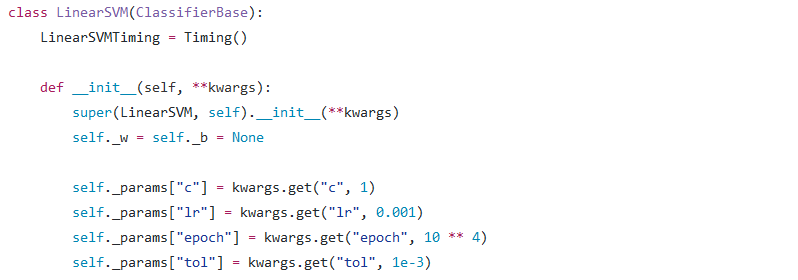（这里是本章用到的 GitHub 地址）

• 输入：• 损失函数：，其中• 输出：• Tensorflow 的核心在于它能构建出一张“运算图（Graph）”，我们需要做的是往这张 Graph 里加入元素
• 基本的元素有如下三种：常量（constant）、可训练的变量（Variable）和不可训练的变量（Variable(trainable=False)）
• 由于机器学习算法常常可以转化为最小化损失函数，Tensorflow 利用这一点、将“最小化损失”这一步进行了很好的封装。具体而言，你只需要在 Graph 里面将损失表达出来后再调用相应的函数、即可完成所有可训练的变量的更新

import tensorflow as tf

# 定义常量、同时把数据类型定义为能够进行 GPU 计算的 tf.float32 类型
x = tf.constant(1, dtype=tf.float32)
# 定义可训练的变量
y = tf.Variable(2, dtype=tf.float32)
# 定义不可训练的变量
z = tf.Variable(3, dtype=tf.float32, trainable=False)
y_sub_z = y – z
x_times_z = x * z
z_div_x = z / x


# 用 Numpy 数组进行 Tensor 的初始化
x = tf.constant(np.array([[1, 2], [3, 4]]))
# Tensorflow 中对应于 np.sum 的方法
axis0 = tf.reduce_sum(x, axis=0)      # 将会得到值为 [ 4 6 ] 的 Tensor
axis1 = tf.reduce_sum(x, axis=1)      # 将会得到值为 [ 3 7 ] 的 Tensor


• Graph 中定义的是一套“运算规则”
• Session 则会“启动”这一套由 Graph 定义的运算规则，而在启动的过程中、Session 可能会额外做三件事：
• 从运算规则中提取出想要的中间结果
• 更新所有可训练的变量（如果启动的运算规则包括“更新参数”这一步的话）
• 赋予“运算规则”中一些“占位符”以具体的值

x = tf.constant(1)
y = x + 1
z = y + 1
print(tf.Session().run(y))   # 将会输出　2

print(tf.Session().run([y, z]))   # 将会输出 [2, 3]


x = tf.Variable(1)
print(tf.Session().run(x))   # 报错！


x = tf.Variable(1)
with tf.Session().as_default() as sess:
sess.run(tf.global_variables_initializer())
print(sess.run(x))

import tensorflow as tf
from Util.Bases import ClassifierBase

class TFLinearSVM(ClassifierBase):
def __init__(self):
super(TFLinearSVM, self).__init__()
self._w = self._b = None
# 使用 self._sess 属性来存储一个 Session 以方便调用
self._sess = tf.Session()

def fit(self, x, y, sample_weight=None, lr=0.001, epoch=10 ** 4, tol=1e-3):
# 将 sample_weight（样本权重）转换为 constant Tensor
if sample_weight is None:
sample_weight = tf.constant(
np.ones(len(y)), dtype=tf.float32, name="sample_weight")
else:
sample_weight = tf.constant(
np.array(sample_weight) * len(y), dtype=tf.float32, name="sample_weight")
# 将输入数据转换为 constant Tensor
x, y = tf.constant(x, dtype=tf.float32), tf.constant(y, dtype=tf.float32)
# 将需要训练的 w、b 定义为可训练 Variable
self._w = tf.Variable(np.zeros(x.shape), dtype=tf.float32, name="w")
self._b = tf.Variable(0., dtype=tf.float32, name="b")
# ========== 接下来的步骤很重要！！！ ==========
# 调用相应方法获得当前模型预测值
y_pred = self.predict(x, True, False)
# 利用相应函数计算出总损失：
# cost = ∑_(i=1)^N max⁡(1-y_i⋅(w⋅x_i+b),0)+1/2 + 0.5 * ‖w‖^2
cost = tf.reduce_sum(tf.maximum(
1 - y * y_pred, 0) * sample_weight) + tf.nn.l2_loss(self._w)
# 利用 Tensorflow 封装好的优化器定义“更新参数”步骤
# 该步骤会调用相应算法、以减少上述总损失为目的来进行参数的更新
# 初始化所有 Variable
self._sess.run(tf.global_variables_initializer())
# 不断调用“更新参数”步骤；如果期间发现误差小于阈值的话就提前终止迭代
for _ in range(epoch):
# 这种写法是比较偷懒的写法，得到的 cost 将不太精确
if self._sess.run([cost, train_step]) < tol:
break


def predict(self, x, get_raw_results=False, out_of_sess=True):
# 利用 reduce_sum 方法算出预测向量
rs = tf.reduce_sum(self._w * x, axis=1) + self._b
if not get_raw_results:
rs = tf.sign(rs)
# 如果 out_of_sess 参数为 True、就要利用 Session 把具体数值算出来
if out_of_sess:
rs = self._sess.run(rs)
# 否则、直接把 Tensor 返回即可
return rs


• Tensorflow 在内部进行 Graph 运算时是无需把具体数值算出来的、不如说使用原生态的 Tensor 进行运算反而会快很多
• 当模型训练完毕后，在测试阶段我们希望得到的当然是具体数值而非 Tensor、此时就需要 Session 帮我们把中间结果提取出来了

# 定义一个数据类型为 tf.float32、“长”未知、“宽”为 2 的矩阵 Placeholder
x = tf.placeholder(tf.float32, [None, 2])
# 定义一个 numpy 数组：[ [ 1 2 ], [ 3 4 ], [ 5 6 ] ]
y = np.array([[1, 2], [3, 4], [5, 6]])
# 定义 x + 1 对应的 Tensor
z = x + 1
# 利用 Session 及其 feed_dict 参数、将 y 的值赋予给 x、同时输出 z 的值
print(tf.Session().run(z, feed_dict={x: y}))   # 将会输出 [ [ 2 3 ], [ 4 5 ], [ 6 7 ] ]

• 把计算损失所涉及的所有 x、y 定义为占位符
• 每次训练时，通过 feed_dict 参数、将原数据的一个小 Batch 赋予给 x、y